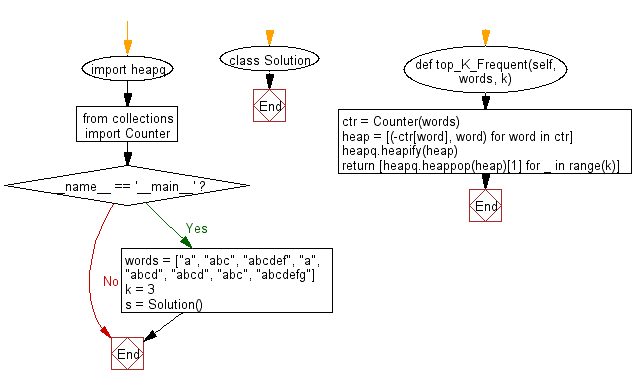﻿ Python heap queue algorithm: Get the k most frequent elements from a given non-empty list of words using Heap queue algorithm - w3resource

# Python: Get the k most frequent elements from a given non-empty list of words using Heap queue algorithm

## Python heap queue algorithm: Exercise-14 with Solution

Write a Python program to get the k most frequent elements from a given non-empty list of words using the heap queue algorithm

Note:
Range of k, 1 ≤ k ≤ number of unique elements and input words contain only lowercase letters.

Sample Solution:

Python Code:

``````import heapq
from collections import Counter
class Solution:
def top_K_Frequent(self, words, k):
"""
:type words: List[str]
:type k: int
:return type: List[str]
"""
ctr = Counter(words)
heap = [(-ctr[word], word) for word in ctr]
heapq.heapify(heap)
return [heapq.heappop(heap) for _ in range(k)]

if __name__ == '__main__':
words = ["a", "abc", "abcdef", "a", "abcd", "abcd", "abc", "abcdefg"]
k = 3
s = Solution()
print("3 most frequent elements:")
print(s.top_K_Frequent(words, k))
```
```

Sample Output:

```3 most frequent elements:
['a', 'abc', 'abcd']
```

Flowchart:Python Code Editor:

Have another way to solve this solution? Contribute your code (and comments) through Disqus.

What is the difficulty level of this exercise?

Test your Programming skills with w3resource's quiz.

﻿